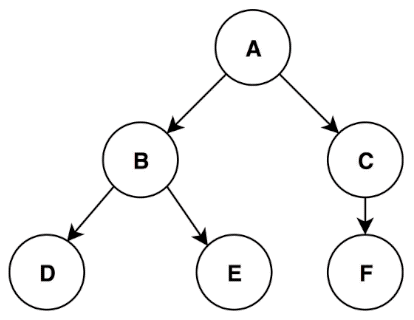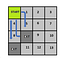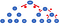# How Depth First Search Algorithm Could Save Your LifeAnimated Depth First Search. (Source: https://dev.to/externconst/dfsubviews-5182)

# Problem Introduction

As we said in the article about Breadth First Search Algorithm, all programming tasks can be expressed through connected graphs and in most of the cases the solution is to traverse the graph according to a specific order.

To explain the BFS Algorithm we assumed that an agent had to escape from a labyrinth as soon as possible. And so there were three possibilities to escape how it is showed:Three choices to escape from the maze

If we try to solve this problem with the help of the graph search, actually we will specifically deal with the case in which starting from a root node the exit is reached by crossing all the nodes at each level of the tree.
For the sake of semplicity we can let the agent move only right and down starting from the first position. In this way we get a binary tree describing each movement done.How Depth First Search works

In this figure, each node indicate the cell and each branch represent the step or better the movement from a cell to another. The DFS (depth first search algorithm) iterations follow the red numbers order.
At the beginning the algorithm searches for the new cells to the right and at the bottom of the current position, subsequently for one of the generated cells (if the end is not reached) it generates other children cells at each level of the tree. Therefore, for each node, its child nodes will be gradually explored before moving on to the exploration of the other nodes belonging to the first level.

# Code

So now we see the pseudocode of the DFS and then we try to implement it on Python.

`DFS-iterative (G, s):                                   //Where G is graph and s is source vertex      let S be stack      S.push( s )            //Inserting s in stack       mark s as visited.      while ( S is not empty):          //Pop a vertex from stack to visit next          v  =  S.top( )         S.pop( )         //Push all the neighbours of v in stack that are not visited           for all neighbours w of v in Graph G:            if w is not visited :                     S.push( w )                             mark w as visited    DFS-recursive(G, s):        mark s as visited        for all neighbours w of s in Graph G:            if w is not visited:                DFS-recursive(G, w)Source: https://www.hackerearth.com/practice/algorithms/graphs/depth-first-search/tutorial/`

To solve our specific problem we adapt the pseudocode in Python, so we get this:

At the beginning we created the matrix that represent our Maze. Then we built the node class of the tree. It is a simple class that build a node with its own value, its own coordinates and moreover it knows its father node.

After setting the margins where the agent cannot exceed (the height and width of the room) and instantiating the root element of the list, we get inside the algorithm cycle. The cycle takes a node from the fringe list (the first node is the root) and then it checks if it is the end of the journey, if it’ s not so new children nodes are generated (corrisponding to the turn right or turn down).
Note that if it finds the end of the path, it creates a list that contains all the nodes that are crossed, simply by recursively identifying all the parent nodes starting from the last node corresponding to the exit.

# Conclusion

We can notice that differently from BFS this time the goal is reached after few steps. This means that DFS (for this specific problem) performs better. Obviously one is better than the other depending on the the task and the time complexity.
BFS is better when target is closer to the source, rather than DFS is better when target is far from the source.

Data Scientist @TIM_Official | Machine learning and Data mining enthusiast

Data Scientist @TIM_Official | Machine learning and Data mining enthusiast